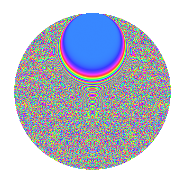# Properties

 Label 3150.2.ebLevel 3150 Weight 2 Character orbit eb Rep. character $$\chi_{3150}(187,\cdot)$$ Character field $$\Q(\zeta_{60})$$ Dimension 3840 Sturm bound 1440

# Related objects

## Defining parameters

 Level: $$N$$ = $$3150 = 2 \cdot 3^{2} \cdot 5^{2} \cdot 7$$ Weight: $$k$$ = $$2$$ Character orbit: $$[\chi]$$ = 3150.eb (of order $$60$$ and degree $$16$$) Character conductor: $$\operatorname{cond}(\chi)$$ = $$1575$$ Character field: $$\Q(\zeta_{60})$$ Sturm bound: $$1440$$

## Dimensions

The following table gives the dimensions of various subspaces of $$M_{2}(3150, [\chi])$$.

Total New Old
Modular forms 11648 3840 7808
Cusp forms 11392 3840 7552
Eisenstein series 256 0 256

## Trace form

 $$3840q + O(q^{10})$$ $$3840q + 12q^{15} - 480q^{16} - 36q^{17} - 16q^{18} + 16q^{23} + 36q^{27} - 12q^{30} + 60q^{33} - 48q^{35} - 40q^{39} - 4q^{42} + 36q^{45} + 16q^{50} + 40q^{53} - 48q^{57} - 24q^{58} - 8q^{60} - 92q^{63} + 8q^{65} + 420q^{69} + 8q^{72} + 240q^{77} + 16q^{78} - 672q^{83} - 120q^{84} + 300q^{89} + 144q^{90} - 8q^{92} + 60q^{93} - 64q^{95} + 48q^{98} + O(q^{100})$$

## Decomposition of $$S_{2}^{\mathrm{new}}(3150, [\chi])$$ into newform subspaces

The newforms in this space have not yet been added to the LMFDB.

## Decomposition of $$S_{2}^{\mathrm{old}}(3150, [\chi])$$ into lower level spaces

$$S_{2}^{\mathrm{old}}(3150, [\chi]) \cong$$ $$S_{2}^{\mathrm{new}}(1575, [\chi])$$$$^{\oplus 2}$$

## Hecke Characteristic Polynomials

There are no characteristic polynomials of Hecke operators in the database